## Introduction

For this vignette we are going to be working with a dataset obtained from the R package spatstat. We will set up a two-dimensional LGCP to estimate Gorilla abundance.

## Setting things up

library(inlabru)
library(INLA)
library(mgcv)
library(ggplot2)
bru_safe_sp(force = TRUE)

## Get the data

For the next few practicals we are going to be working with a dataset obtained from the R package spatstat, which contains the locations of 647 gorilla nests. We load the dataset like this:

data(gorillas, package = "inlabru")

This dataset is a list containing a number of R objects, including the locations of the nests, the boundary of the survey area and an INLA mesh - see help(gorillas) for details. Extract the the objects we need from the list, into other objects, so that we don’t have to keep typing ‘gorillas$’: nests <- gorillas$nests
mesh <- gorillas$mesh boundary <- gorillas$boundary

Plot the points (the nests).

ggplot() +
geom_fm(data = mesh) +
gg(nests) +
gg(boundary, fill = "red", alpha = 0.2) +
ggtitle("Points")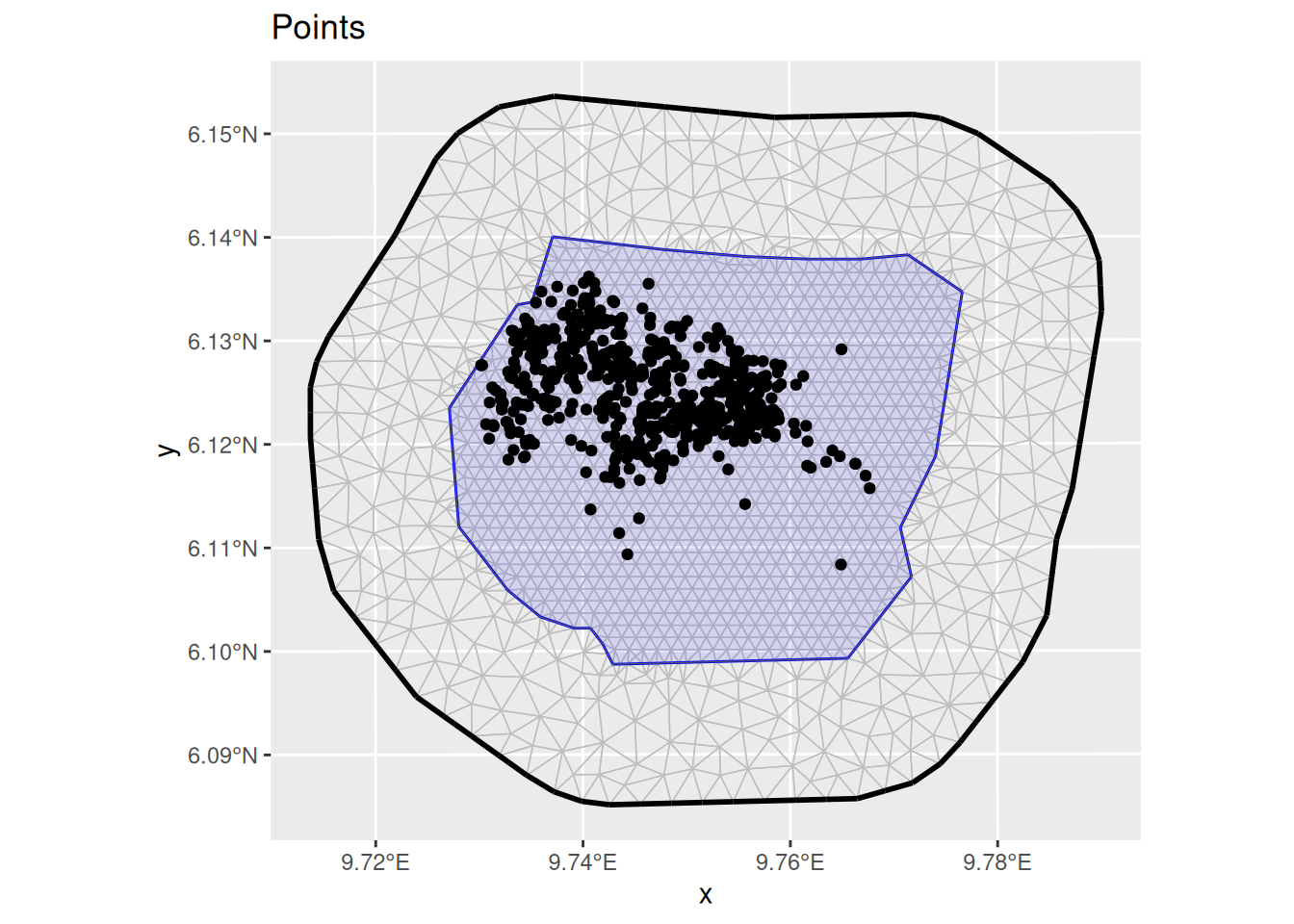## Fiting the model

Fit an LGCP model to the locations of the gorilla nests, predict on the survey region, and produce a plot of the estimated density - which should look like the plot shown below.

Recall that the steps to specifying, fitting and predicting are:

1. Specify a model, comprising (for 2D models) coordinates on the left of ~ and an SPDE + Intercept(1) on the right. Please use the SPDE prior specification stated below.

2. Call lgcp( ), passing it (with 2D models) the model components, the SpatialPointsDataFrame containing the observed points and the SpatialPolygonsDataFrame defining the survey boundary using the samplers argument.

3. Call predict( ), passing it the fitted model from 2., locations at which to predict and an appropriate predictor specification. The locations at which to predict can be a SpatialPixelsDataFrame covering the mesh, obtained by calling fm_pixels(mesh, format = "sp").

matern <- inla.spde2.pcmatern(
mesh,
prior.sigma = c(0.1, 0.01),
prior.range = c(5, 0.01)
)

cmp <- coordinates ~
mySmooth(coordinates, model = matern) +
Intercept(1)

fit <- lgcp(cmp, nests, samplers = boundary, domain = list(coordinates = mesh))

## Predicting intensity

You should get a plot like that below (the command below assumes that the prediction is in an object called lambda):

pred <- predict(
fit,
fm_pixels(mesh, mask = boundary, format = "sp"),
~ data.frame(
lambda = exp(mySmooth + Intercept),
loglambda = mySmooth + Intercept
)
)

pl1 <- ggplot() +
gg(pred$lambda) + gg(boundary) + ggtitle("LGCP fit to Points", subtitle = "(Response Scale)") pl2 <- ggplot() + gg(pred$loglambda) +
gg(boundary, alpha = 0) +
ggtitle("LGCP fit to Points", subtitle = "(Linear Predictor Scale)")

multiplot(pl1, pl2, cols = 2)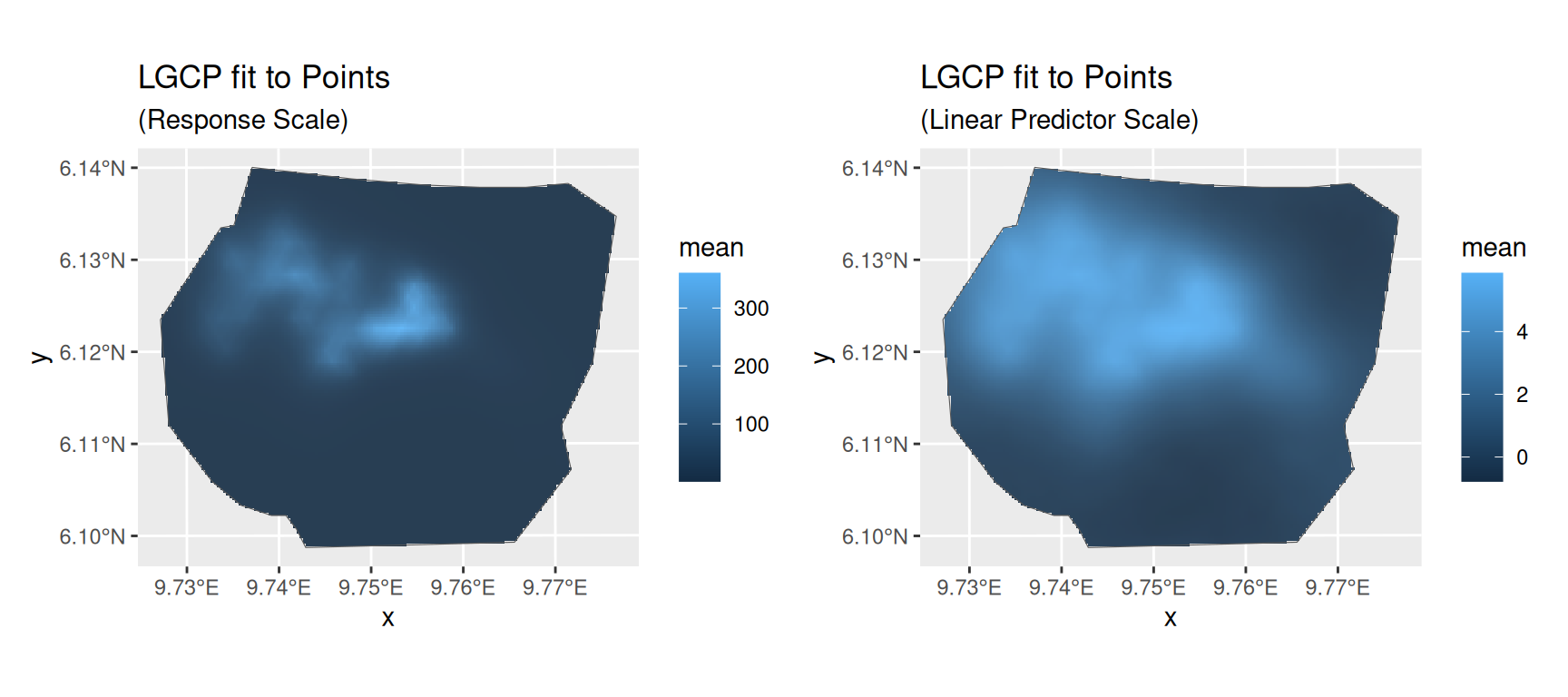You can plot the median, lower 95% and upper 95% density surfaces as follows (assuming that the predicted intensity is in object lambda).

ggplot() +
gg(cbind(pred$lambda, data.frame(property = "q0.500")), aes(fill = median)) + gg(cbind(pred$lambda, data.frame(property = "q0.025")), aes(fill = q0.025)) +
gg(cbind(pred$lambda, data.frame(property = "q0.975")), aes(fill = q0.975)) + coord_equal() + facet_wrap(~property)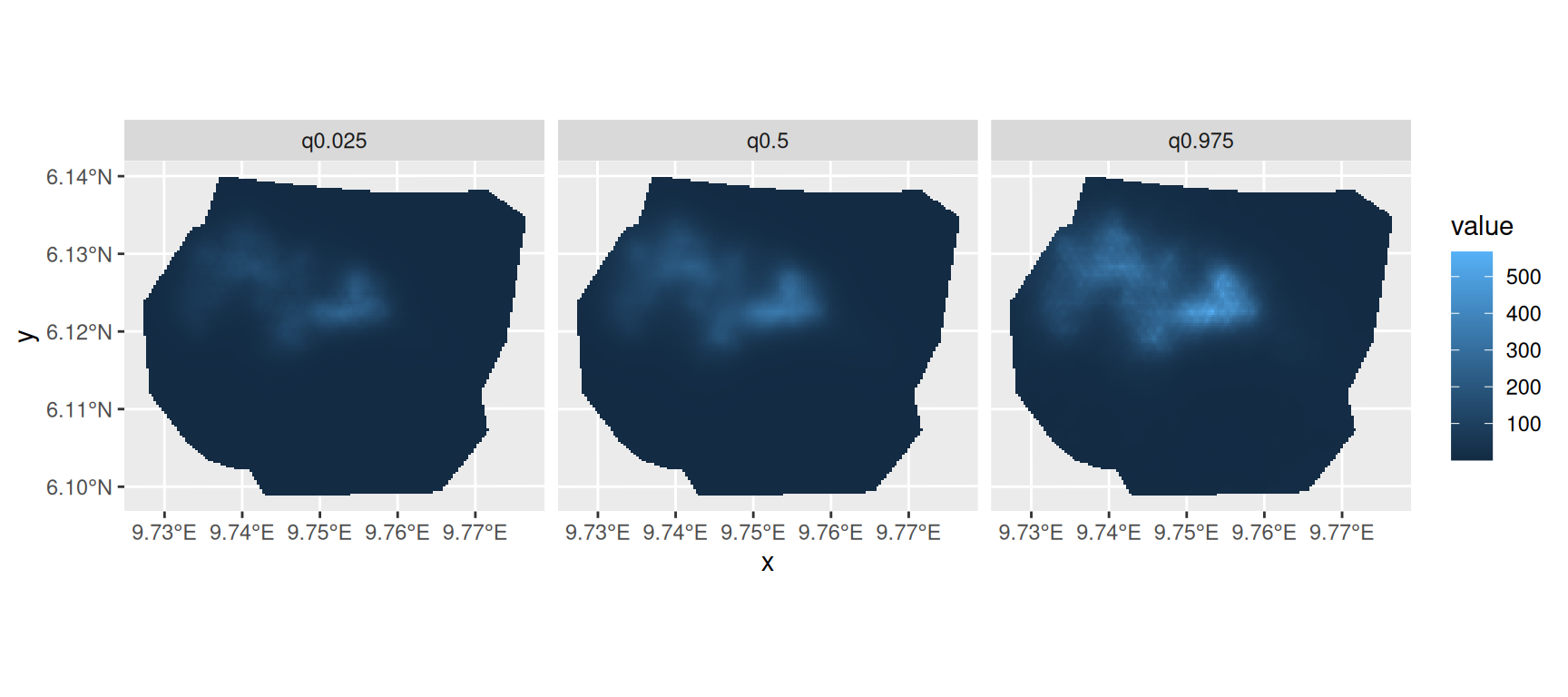## SPDE parameters Plot the SPDE parameter and fixed effect parameter posteriors. int.plot <- plot(fit, "Intercept") spde.range <- spde.posterior(fit, "mySmooth", what = "range") spde.logvar <- spde.posterior(fit, "mySmooth", what = "log.variance") range.plot <- plot(spde.range) var.plot <- plot(spde.logvar) multiplot(range.plot, var.plot, int.plot)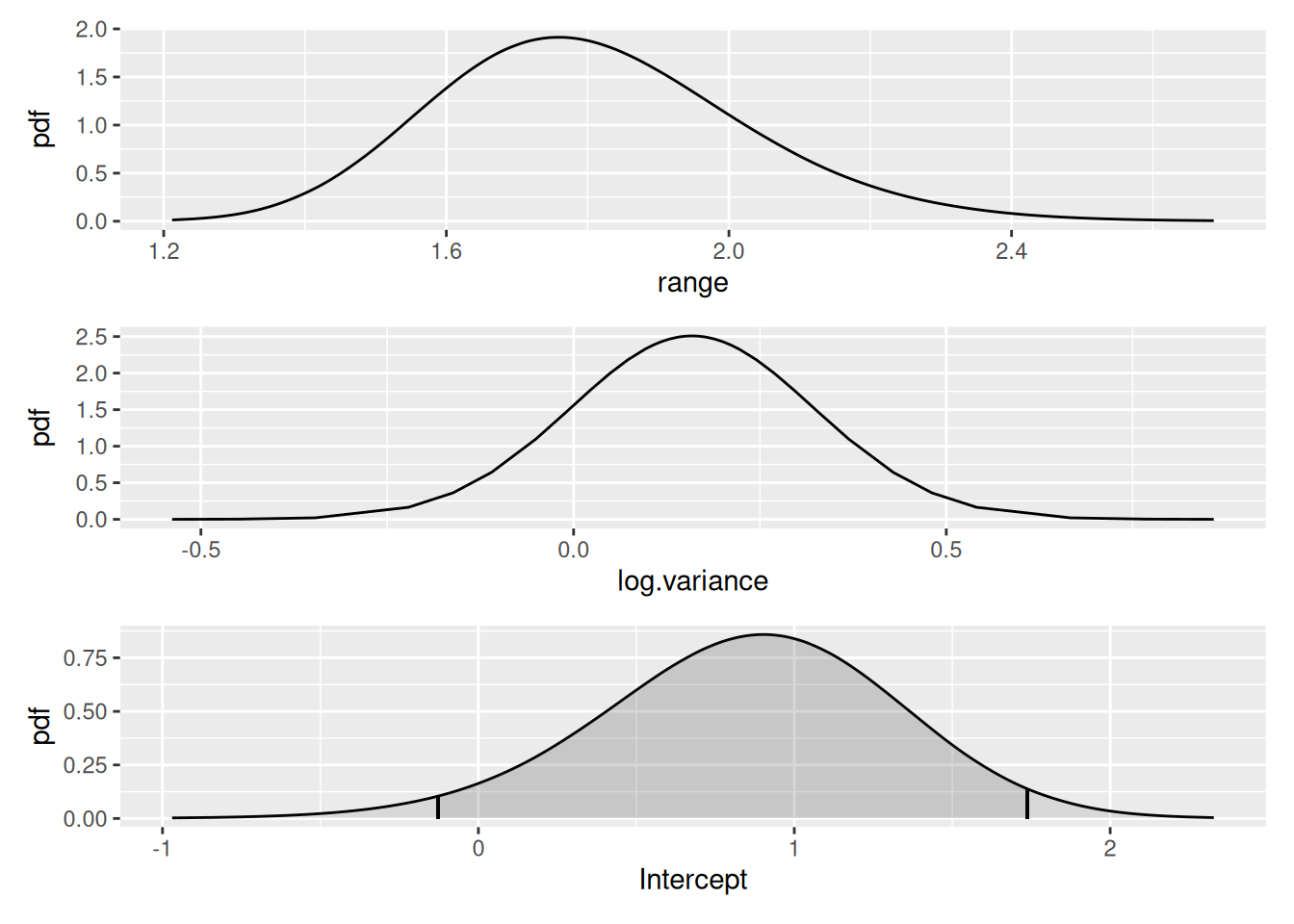Look at the correlation function if you want to: corplot <- plot(spde.posterior(fit, "mySmooth", what = "matern.correlation")) covplot <- plot(spde.posterior(fit, "mySmooth", what = "matern.covariance")) multiplot(covplot, corplot)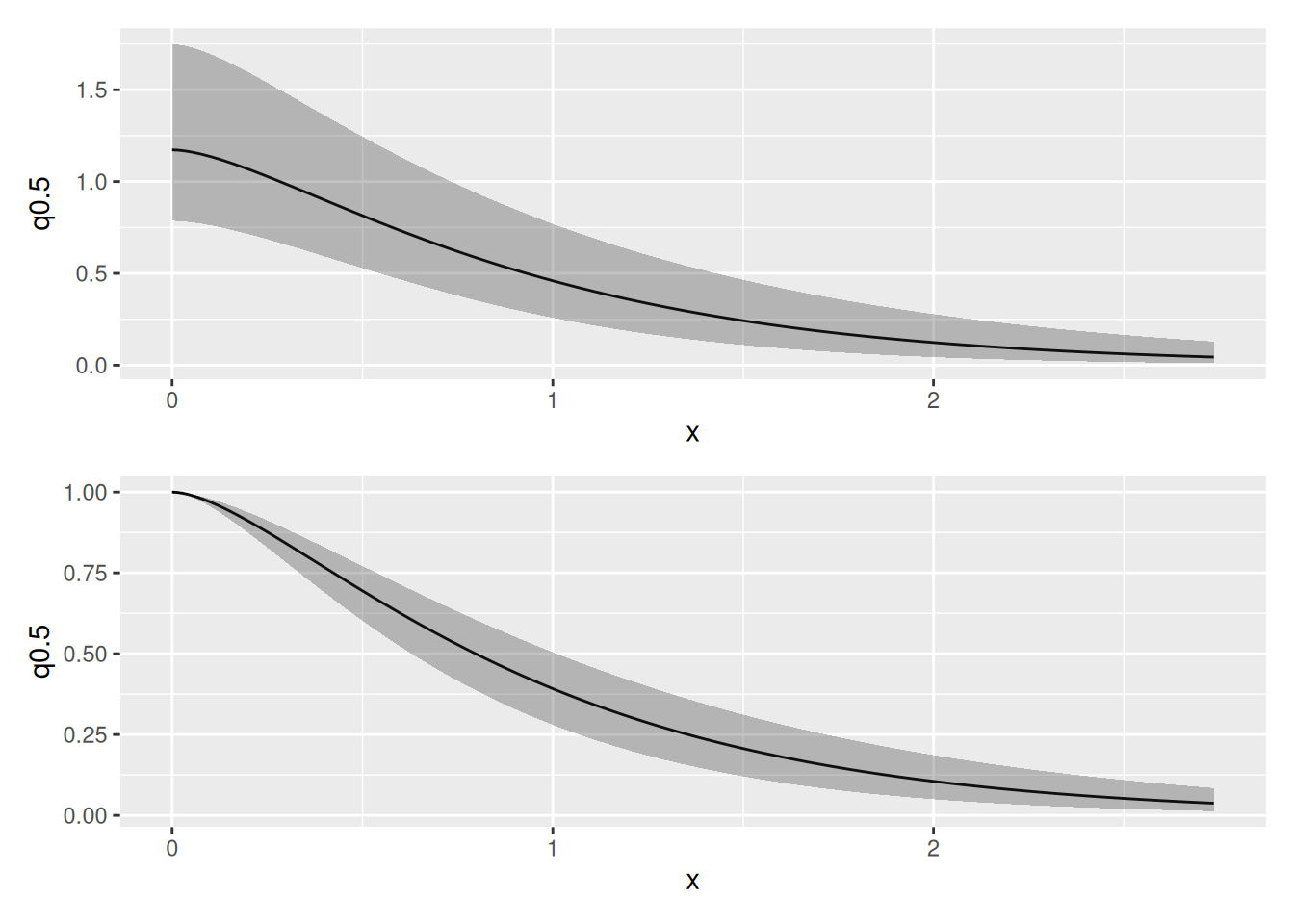## Estimating Abundance Finally, estimate abundance using the predict function. As a first step we need an estimate for the integrated lambda. The integration weight values are contained in the fm_int() output. Lambda <- predict( fit, fm_int(mesh, boundary), ~ sum(weight * exp(mySmooth + Intercept)) ) Lambda #> mean sd q0.025 q0.5 q0.975 median mean.mc_std_err #> 1 670.0177 30.3882 614.7392 671.14 729.3213 671.14 3.03882 #> sd.mc_std_err #> 1 2.050789 Given some generous interval boundaries (500, 800) for lambda we can estimate the posterior abundance distribution via Nest <- predict( fit, fm_int(mesh, boundary), ~ data.frame( N = 500:800, dpois(500:800, lambda = sum(weight * exp(mySmooth + Intercept)) ) ) ) Get its quantiles via inla.qmarginal(c(0.025, 0.5, 0.975), marginal = list(x = Nest$N, y = Nest$mean)) #>  599.8821 673.8480 753.1823 … the mean via inla.emarginal(identity, marginal = list(x = Nest$N, y = Nest$mean)) #>  674.4301 and plot posteriors: Nest$plugin_estimate <- dpois(Nest$N, lambda = Lambda$mean)
ggplot(data = Nest) +
geom_line(aes(x = N, y = mean, colour = "Posterior")) +
geom_line(aes(x = N, y = plugin_estimate, colour = "Plugin"))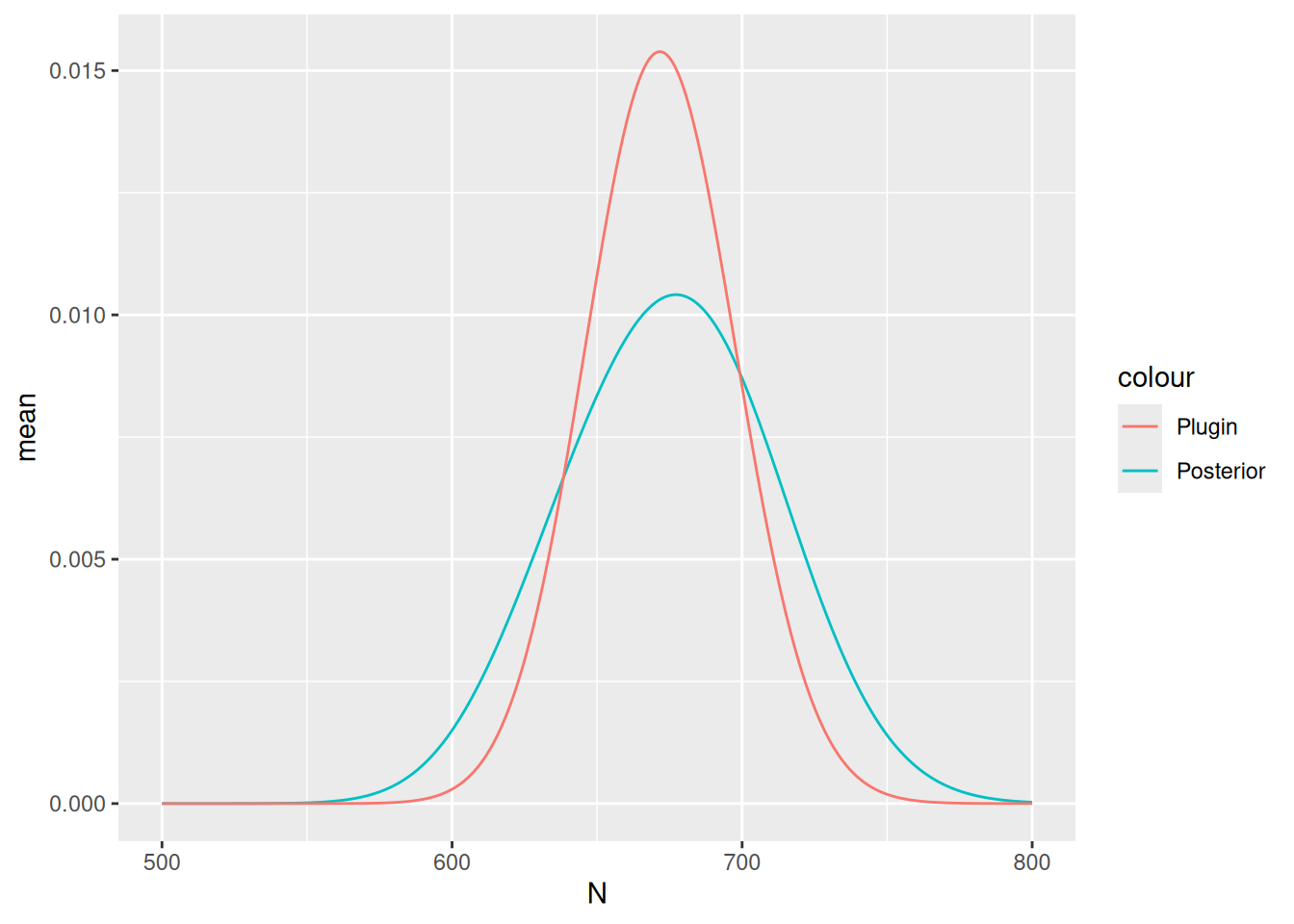The true number of nests in 647; the mean and median of the posterior distribution of abundance should be close to this if you have not done anything wrong!